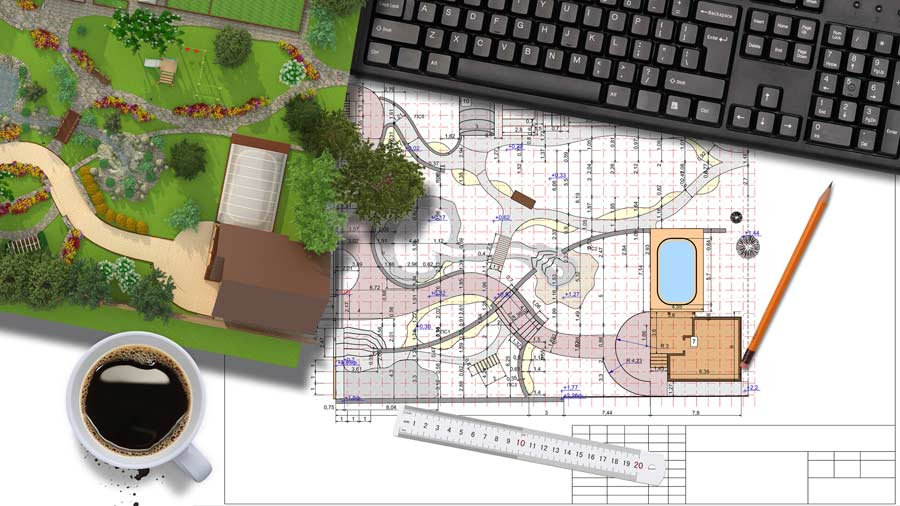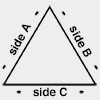Calc

# Square Footage Calculator

Use this square footage calculator to work out an area of square feet, square meters or square yards for flooring, landscaping, carpets, tiling or construction projects. This tool also provides a cost of materials estimate.

ft in
ft in
ft in
ft in
ft in

#### Disclaimer

Whilst every effort has been made in building this square footage calculator, we are not to be held liable for any special, incidental, indirect or consequential damages or monetary losses of any kind arising out of or in connection with the use of the converter tools and information derived from the web site. This square footage calculator is here purely as a service to you, please use it at your own risk. Do not use calculations for anything where loss of life, money, property, etc could result from inaccurate conversions.

## How do I calculate square feet?

Let's say you have a rectangular-shaped room and you want to calculate the square footage area for flooring or carpet. The easiest method for calculating the square footage is to measure the length and width in feet and then multiply the two figures together to give you a result in ft2. If you're measuring a room for flooring, take a look at our article on how to measure for a new floor.

Should you find yourself needing to calculate an area of square feet in an 'L' shape, consider dividing the shape up into rectangular sections and treating them as separate areas for calculation (adding them together at the end).

### Calculating square footage of your area

2. If your measurements aren't in feet, convert them to feet (converter available):
• If in inches: divide each by 12.
• If in yards: multiply each by 3.
• If in cm: multiply each by 0.03281.
• If in m: multiply each by 3.281.
3. Multiply your Length × Width = Area.

This gives you your area in square feet (ft2). To work out your cost of materials, simply multiply this figure by your 'cost per square feet'. For other area shapes (circles, triangles), instructions and formulae are below. Should you wish to convert to acres, give the square feet to acres converter a try. If you would like to convert your figure into cubic feet, take a look at the square feet to cubic feet calculator.

Of course, it may be that the area you're measuring isn't rectangular. Instructions on calculating for a circular area and triangular area are further down.### How many square feet are in a 10x10 room?

To answer this question, we simply multiply the length of the room by the width. If your room is 10 feet long by 10 feet wide, 10 × 10 = 100 square feet.

## How to calculate the square footage of a house - example

Supposing you want to measure the square footage of an area that isn't one single rectangle? This example video, from Mathispower4u, shows you how to divide your area up into smaller rectangles in order to calculate the overall square footage.

### Calculating square footage for a circular area1. Measure the diameter of your circle in feet.
2. To calculate the area of a circle we use the formula: π x (diameter/2)2. That is to say π (pi is 3.14159265) multiplied by half the diameter squared. So, we divide our diameter by 2 and then square it (multiply it by itself) and then multiply by π.
3. This gives you your square feet figure (ft2). To work out your cost of materials, simply multiply this figure by your 'cost per square feet'.

The formula for calculating the area of a circle is: π r2 (with r being the radius of the circle, which is half the diameter). π is the symbol for pi (3.14159265).

### Calculating square footage for a triangular area1. Measure the length of the base of the triangle and the height of the triangle.
2. To calculate the area of a triangle we use the formula: (base x height) / 2. That is to say we multiply the base by the height and then divide it by 2.
3. This gives you your square feet figure (ft2). To work out your cost of materials, simply multiply this figure by your 'cost per square feet'.

The formula for calculating the square footage area of a triangle shape is: base x height / 2.